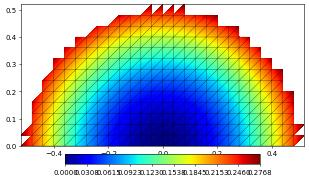# FilteredGridView examples

In this example we use filteredGridView to filter out parts of a given grid view.

:

from ufl import SpatialCoordinate, dot
from dune.grid import cartesianDomain
from dune.alugrid import aluConformGrid as leafGridView
from dune.fem.view import filteredGridView
from dune.fem.space import lagrange


Create a host grid view of the underlying grid as usual.

:

gridView = leafGridView( cartesianDomain([0,0],[1,1],[16,16]) )


Now create a filteredGridView with a simple callable specifying which element belongs to the new domain.

:

filteredView = filteredGridView(gridView, lambda e: e.geometry.center.two_norm > 0.5, domainId=1)
space = lagrange(filteredView, order=2)
x = SpatialCoordinate(space)
solution = space.interpolate(dot(x,x),name="solution")
solution.plot()
print("number of dofs:", solution.size,\
"integral over filtered domain",solution.integrate())number of dofs: 1089 integral over filtered domain 0.6413014729817705


Revert the filter and create a filteredGridView with also provides an overloaded index set with a consecutive index for the entities belonging to the new domain.

:

filteredView = filteredGridView(gridView, lambda e: e.geometry.center.two_norm < 0.5, domainId=1,
useFilteredIndexSet=True)
space = lagrange(filteredView, order=2)
x = SpatialCoordinate(space)
solution = space.interpolate(dot(x,x),name="solution")
solution.plot()
print("number of dofs:", solution.size,\
"integral over filtered domain",solution.integrate())number of dofs: 239 integral over filtered domain 0.025365193684895822


Compare with the original grid view.

:

space = lagrange(gridView, order=2)
solution = space.interpolate(dot(x,x),name="solution")
solution.plot()
print("number of dofs:", solution.size,\
"integral over filtered domain",solution.integrate())number of dofs: 1089 integral over filtered domain 0.6666666666666667


This page was generated from the notebook filteredgridview_nb.ipynb and is part of the tutorial for the dune-fem python bindings